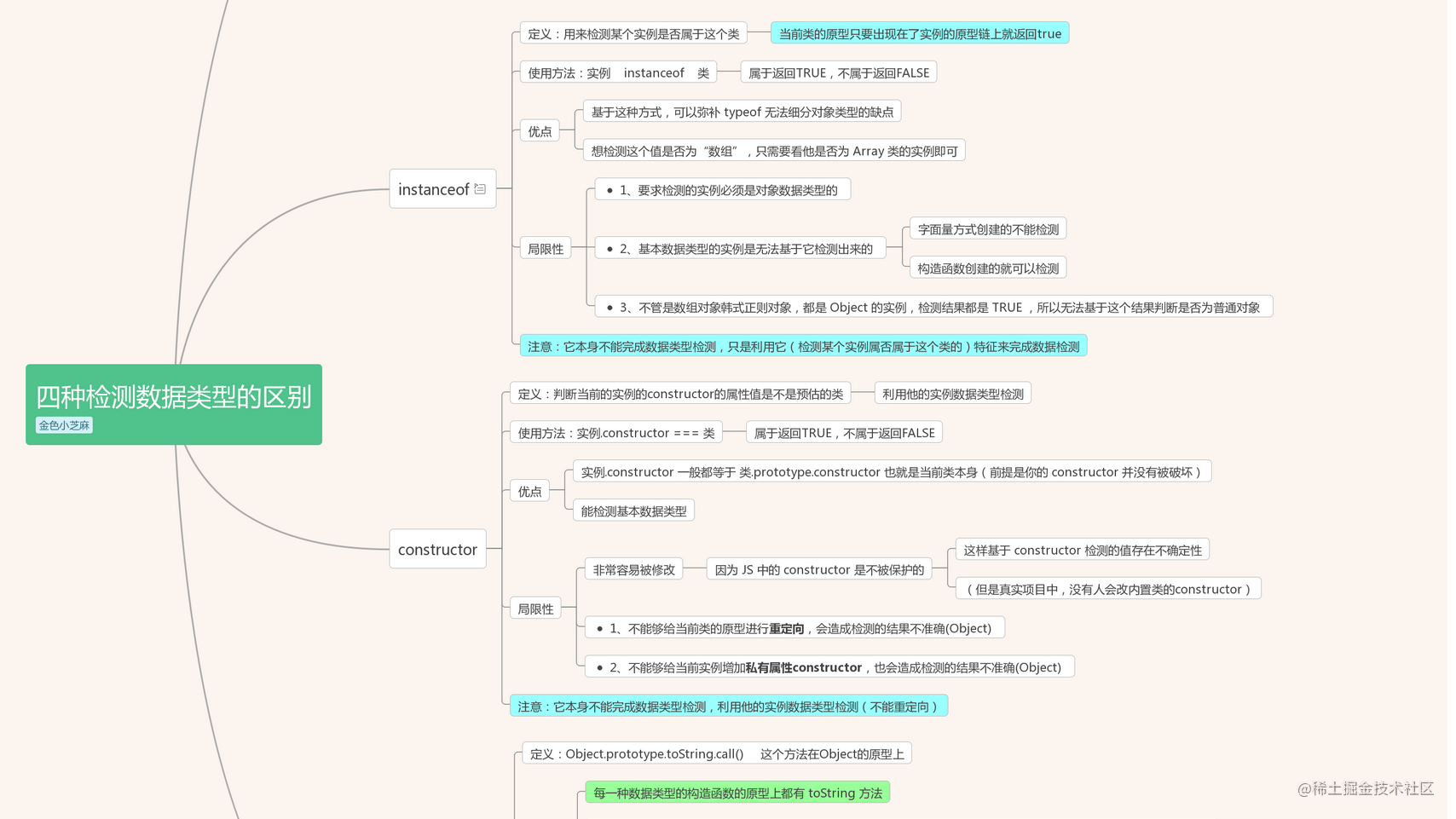# JS中数据类型检测四种方式的优缺点

• `tyepof [value]` ：检测数据类型的运算符
• `[example] instanceof [class]` ： 检测某一个实例是否属于这个类
• `[example].constructor===[class]` ：检测实例和类关系的，从而检测数据类型
• `Object.prototype.toString.call([value])`：检测数据类型

# 一、typeof

• 1、定义：用来检测数据类型的运算符
• 2、语法：`tyepof [value]`
• 3、返回值：
• `typeof` 检测的结果首先是一个字符串；
• 字符串中包含了对应的数据类型（例如： `“number”``“string”``“boolean”``“undefined”``“object”``“function”``“symbol”``“bigint”`

• 4、优点：使用起来简单，基本数据类型值基本上都可以有效检测，引用数据类型值也可以检测出来
• 5、局限性（特殊值）
• 1)、`NaN` / `Infinity` 都是数字类型的，检测结果都是`“number”`;
• 2)、`typeof null` 的结果是`“object”`;
• （这是浏览器的`BUG`：所有的值在计算中都以二进制编码储存，浏览器中把前三位`000`的当作对象，而`null`的二进制前三位是`000`，所以被识别为对象，但是他不是对象，他是空对象指针，是基本类型值）
• 3)、`typeof` `普通对象/数组对象/正则对象...`， 结果都是`“object”`，这样就无法基于`typeof` 区分是`普通对象`还是`数组对象``...`等了
• 6、应用场景
• 已知有一个变量x，但是我们无法确认其数据类型，我们需要有一个判断操作：当x的类型是对象的时候（什么对象都可以），则处理对应的事情
``````if (typeof x == "object") {
//=>null检测结果也会是"object"，所以结果是"object"不一定是对象，还可能是null呢
...
}

``````if (x != null && typeof x == "object") {
// ...
}

• 7、练习题

console.log(typeof []); //=>"object"

console.log(typeof typeof typeof []); //=>"string"

# 二、instanceof

• 1、定义：用来检测某个实例是否属于这个类
• 当前类的原型只要出现在了实例的原型链上就返回`true`
• 2、语法：`实例 instanceof 类`
• 3、属于返回`TRUE`，不属于返回`FALSE`
• 4、优点：
• 基于这种方式，可以弥补 `typeof` 无法细分对象类型的缺点（想检测这个值是否为数组，只需要看他是否为Array类的实例即可）
``````let arr = [10, 20];

console.log(typeof arr); //=>"object"
console.log(arr instanceof Array); //=>true
console.log(arr instanceof RegExp); //=>false
console.log(arr instanceof Object); //=>true  不管是数组对象还是正则对象，都是Object的实例，检测结果都是TRUE，所以无法基于这个结果判断是否为普通对象

• 5、局限性:
• 1)、要求检测的实例必须是对象数据类型的
• 2)、基本数据类型的实例是无法基于它检测出来的
• 字面量方式创建的不能检测
• 构造函数创建的就可以检测
• 3)、不管是数组对象韩式正则对象，都是 Object 的实例，检测结果都是 TRUE ，所以无法基于这个结果判断是否为普通对象
``````// instanceof检测的实例必须都是引用数据类型的，它对基本数据类型值操作无效
console.log(10 instanceof Number); //=>false
console.log(new Number(10) instanceof Number); //=>true

// instanceof检测机制：验证当前类的原型prototype是否会出现在实例的原型链__proto__上，只要在它的原型链上，则结果都为TRUE
function Fn() {}
Fn.prototype = Object.create(Array.prototype);
let f = new Fn;
console.log(f instanceof Array); //=>true f其实不是数组，因为它连数组的基本结构都是不具备的

# 三、constructor

• 1、定义：判断当前的实例的`constructor`的属性值是不是预估的类（利用他的实例数据类型检测）
• 2、语法：`实例.constructor === 类`
• 3、返回值：属于返回`TRUE`，不属于返回`FALSE`
• 4、优点：
• `实例.constructor` 一般都等于 `类.prototype.constructor` 也就是当前类本身（前提是你的 `constructor` 并没有被破坏）
• 能检测基本数据类型
• 5、局限性：
• 1)、不能够给当前类的原型进行重定向，会造成检测的结果不准确(`Object`)
• 2)、不能够给当前实例增加私有属性`constructor`，也会造成检测的结果不准确(`Object`)
• 3)、非常容易被修改，因为`JS`中的`constructor`是不被保护的（用户可以自己随便改），这样基于`constructor`检测的值存在不确定性（但是项目中，基本没有人会改内置类的`constructor`
``````let arr = [],
obj = {},
num = 10;
console.log(arr.constructor === Array); //=>true
console.log(arr.constructor === Object); //=>false
console.log(obj.constructor === Object); //=>true
console.log(num.constructor === Number); //=>true

# 四、Object.prototype.toString.call()

• 1、定义：找到`Object.prototype`上的`toString`方法，让`toString`方法执行，并且基于`call`让方法中的`this`指向检测的数据值，这样就可以实现数据类型检测了
• 2、原理：
• 1.每一种数据类型的构造函数的原型上都有`toString`方法；
• 2.除了`Object.prototype`上的`toString`是用来返回当前实例所属类的信息（检测数据类型的），其余的都是转换为字符串的
• 3.`对象实例.toString()``toString`方法中的`THIS`是对象实例，也就是检测它的数据类型，也就是`THIS`是谁，就是检测谁的数据类型
• 4.`Object.prototype.toString.call([value])` 所以我们是把`toString`执行，基于`call`改变`this`为要检测的数据值
• 3、使用方法:
• Object.prototype.toString.call(被检测的实例)
• ({}).toString.call(被检测的实例)
``````Object.prototype.toString.call(10)
({}).toString.call(10)
({}).toString===Object.prototype.toString

• 4、返回值：
• 是一个字符串“[Object 当前被检测实例所属的类]”
``````let class2type = {};
let toString = class2type.toString; //=>Object.prototype.toString

console.log(toString.call(10)); //=>"[object Number]"
console.log(toString.call(NaN)); //=>"[object Number]"
console.log(toString.call("xxx")); //=>"[object String]"
console.log(toString.call(true)); //=>"[object Boolean]"
console.log(toString.call(null)); //=>"[object Null]"
console.log(toString.call(undefined)); //=>"[object Undefined]"
console.log(toString.call(Symbol())); //=>"[object Symbol]"
console.log(toString.call(BigInt(10))); //=>"[object BigInt]"
console.log(toString.call({xxx:'xxx'})); //=>"[object Object]"
console.log(toString.call([10,20])); //=>"[object Array]"
console.log(toString.call(/^\d+\$/)); //=>"[object RegExp]"
console.log(toString.call(function(){})); //=>"[object Function]"

• 5、优点：
• 专门用来检测数据类型的方法，基本上不存在局限性的数据类型检测方式
• 基于他可以有效的检测任何数据类型的值
• 6、局限性：
• 1)、只能检测内置类，不能检测自定义类
• 2)、只要是自定义类返回的都是‘[Object Object]’

# JQ 中基于数据类型检测的方法——自己封装

JQ 中对于数据类型检测，笔者理解的主要还是利用`Object.prototype.toString.call()`方法，也可以说是`Object.prototype.toString.call()`可以完成数据类型检测的原理解析；

``````function toType(obj) {
let class2type = {},
toString = class2type.toString, //=>Object.prototype.toString 检测数据类型
arr = "Boolean Number String Function Array Date RegExp Object Error Symbol".split(" ");
arr.forEach(item => {
class2type["[object " + item + "]"] = item.toLowerCase();
})
/*
console.log(class2type);
{
[object Boolean]: "boolean",
[object Number]: "number",
[object String]: "string"
......
}
*/

//传递给我的是null/undefined，直接返回 "null"/"undefined"
if (obj == null) {
return "" + obj;
}

// typeof obj === "object" || typeof obj === "function" =>引用数据类型
//   => 如果是基本数据类型值，检测数据类型使用typeof就可以
//   => 如果是引用数据类型值，则基于对象的toString就可以
//         => toString.call(obj)  检测当前值的数据类型 "[object Xxx]"
//         => class2type["[object Xxx]"] 当上一步生成的对象中，基于对应的属性名，找到属性值（所属的数据类型），如果没有则返回 "object"
return typeof obj === "object" || typeof obj === "function" ? class2type[toString.call(obj)] || "object" : typeof obj;
};

console.log(toType(1)); //=>"number"
console.log(toType(NaN)); //=>"number"
console.log(toType([])); //=>"array"
console.log(toType(/^\d+\$/)); //=>"regexp"
console.log(toType({})); //=>"object"
console.log(toType(null)); //=>"object"
console.log(toType()); //=>"object"

# 散知识：`Object`原型上的`valueOf`方法

### 基本数据类型中：

• valueOf：是获取原始值 [[PrimitiveValue]]
• toString：是将原始值转换为字符串
``````let num1 = 10,
num2 = new Number(10);

console.log(num1);//=> 10
console.log(num1.valueOf());//=> 10
console.log(num1.toString());//=> "10"

console.log(num2);//=>Number {10}
console.log(num2.valueOf());//=> 10
console.log(num2.toString());//=> "10"

### 引用数据类型中

• 数组中：

``````let arr = [10, 20],
obj = {
xxx: 'xxx'
};

console.log(arr);
console.log(arr.valueOf()); //=>调取的是 Object.prototype.valueOf 原始值
console.log(arr.toString()); //=>调取的是 Array.prototype.toString 转换字符串

console.log(obj);
console.log(obj.valueOf()); //=>调取的是 Object.prototype.valueOf  原始值
console.log(obj.toString()); //=>调取的是 Object.prototype.toString  检测数据类型

### 应用——隐性转换

``````let num1 = 10,
num2 = new Number(10);
let arr = [10, 20],
obj = {
xxx: 'xxx'
};

• =>num1.valueOf() 先获取原始值
• =>[[PrimitiveValue]].toString() 把获取的原始值转换为字符串
• 利用这个机制我们可以做出一道面试题

### 面试题：a == 1 && a == 2 && a == 3

``````//=> 下面代码a在什么值情况下会输出1

var a = ?;
if (a == 1 && a == 2 && a == 3) {
console.log(1);
}

``````var a = {
n : 0,
valueOf(){
return ++this.n;
}
};
if (a == 1 && a == 2 && a == 3) {
console.log(1);
}